## Big Data Hadoop and Spark Developer

Certification Training
29200 Learners
13 Chapters +

# Spark Algorithm Tutorial

Welcome to the fifteenth lesson ‘Spark Algorithm’ of Big Data Hadoop Tutorial which is a part of ‘Big Data Hadoop and Spark Developer Certification course’ offered by Simplilearn.

In this lesson, you will learn about the kinds of processing and analysis that Spark supports. You will also learn about Spark machine learning and its applications, and how GraphX and MLlib work with Spark.

Let us look at the adjectives of this lesson in the next section.

## Objectives

After completing this lesson, you will be able to:

• Describe the kinds of processing and analysis that Spark supports

• Describe the applications of Spark machine learning

• Explain how GraphX and MLlib work with Spark.

In the first topic of the lesson, you will learn about Spark as an iterative algorithm.

## When does Spark work best?

Spark is an open-source cluster-computing framework and provides up to 100 times faster performance for a few applications with in-memory primitives as compared to the disk-based, two-stage MapReduce of Hadoop.

Fast performance makes it suitable for Apache Spark machine learning algorithms, as it allows programs to load data into the memory of a cluster and query the data constantly.

Spark works best in the following use cases:

• There is a large amount of data that requires distributed storage

• There is intensive computation that requires distributed computing

• There are instances where iterative algorithms are present that requires in-memory processing and pipelining.

## Uses of Apache Spark

Here are some examples where Spark is beneficial:

• Spark helps you answer the question for risk analysis, “How likely is it that this borrower will pay back a loan?”

• Spark can answer questions on recommendation such as, “Which products will this customer enjoy?”

• It can help predict events by answering questions such as, “How can we prevent service outages instead of simply reacting to them?”

• Spark helps to classify by answering the question, “How can we tell which mail is spam and which is legitimate?”

With these examples in mind, let’s delve more into the world of Spark. In the following sections, you will learn about how an iterative algorithm runs in Spark.

## Spark: Iterative algorithm

Spark is designed for systems that are required to implement an iterative algorithm.

Let’s look at PageRank Algorithm, which is an iterative algorithm.

### PageRank Algorithm: FEATURES

PageRank is an example of an iterative algorithm. It is one of the methods used to determine the relevance or importance of a webpage. It gives web pages a ranking score based on links from other pages.

A higher rank is given when the links are from many pages, and when the links are from high ranked pages. The algorithm outputs a probability distribution used to represent the likelihood that a person clicking on the links will arrive at a particular page.

PageRank is important because it is a classic example of big data analysis, like WordCount. As there is a lot of data, an algorithm is required that is distributable and scalable.

PageRank is iterative, which means the more the iteration, the better is the answer.

Let’s look at how the PageRank algorithm works.

### PageRank Algorithm: WORKING

Start each page with a rank of 1.0. On each iteration, a page contributes to its neighbors its own rank divided by the number of its neighbors.

Here is the function:

contribp = rankp / neighborsp

You can then set each page’s new rank based on the sum of its neighbors contribution. The function is given here:

new-rank = Σcontribs * .85 + .15

Each iteration incrementally improves the page ranking as shown in the below diagram.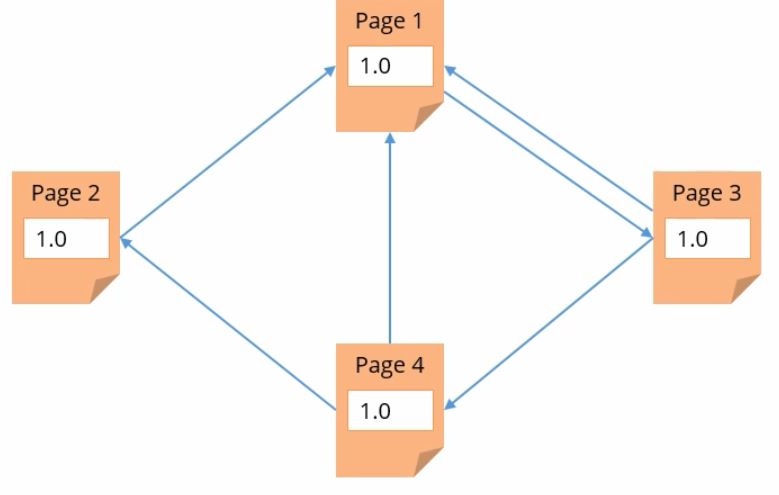In the next section, you will learn about graph-parallel system.

## Graph Parallel System

Today, big graphs exist in various important applications, be it web, advertising, or social networks. Examples of a few of such graphs are presented on the sections.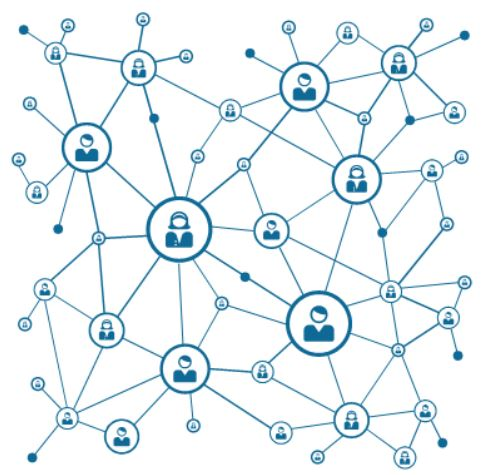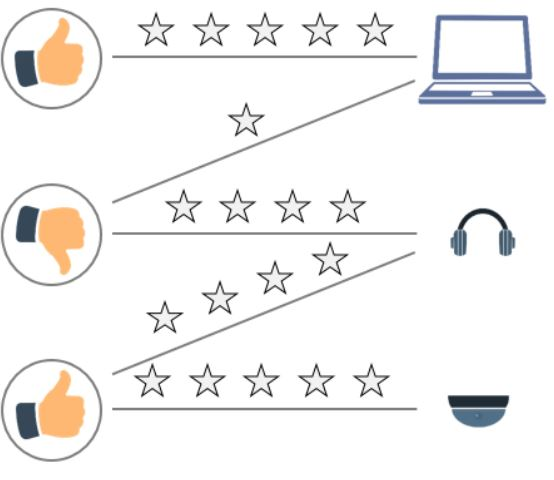These graphs allow the users to perform tasks such as target advertising, identify communities, and decipher the meaning of documents. This is possible by modeling the relations between products, users, and ideas.

As the size and significance of graph data is growing, various new large-scale distributed graph-parallel frameworks, such as GraphLab, Giraph, and PowerGraph, have been developed. With each framework, a new programming abstraction is available. These abstractions allow the users to explain graph algorithms in a compact manner.

They also explain the related runtime engine that can execute these algorithms efficiently on distributed and multicore systems. Additionally, these frameworks abstract the issues of the large-scale distributed system design.

Therefore, they are capable of simplifying the design, application, and implementation of the new sophisticated graph algorithms to large-scale real-world graph problems.

Let’s look at a few limitations of the Graph-parallel system.

## Limitations of Graph-Parallel System

Firstly, although the current graph-parallel system frameworks have various common properties, each of them presents different graph computations. These computations are custom-made for a specific graph applications and algorithms family or the original domain.

Secondly, all these frameworks depend on different runtime. Therefore, it is tricky to create these programming abstractions.

And finally, these frameworks cannot resolve the data Extract, Transform, Load or ETL issues and issues related to the process of deciphering and applying the computation results.

The new graph-parallel system frameworks, however, have built-in support available for interactive graph computation.

Next, you will learn about Spark GraphX.

## What is Spark GraphX?

Spark GraphX is a graph computation system running in the framework of the data parallel system which focuses on distributing the data across different nodes that operate on the data in parallel.

• It addresses the limitations posed by the graph parallel system.

• Spark GraphX is more of a real-time processing framework for the data that can be represented in a graph form.

• Spark GraphX extends the Resilient Distributed Dataset or RDD abstraction and hence, introduces a new feature called Resilient Distributed Graph or RDG.

• Spark GraphX simplifies the graph ETL and analysis process substantially by providing new operations for viewing, filtering, and transforming graphs.

### Features of Spark GraphX

Spark GraphX has many features like:

• Spark GraphX combines the benefits of graph parallel and data parallel systems as it efficiently expresses graph computations within the framework of the data parallel system.

• Spark GraphX distributes graphs efficiently as tabular data structures by leveraging new ideas in their representations.

• It uses in-memory computation and fault tolerance by leveraging the improvements of the data flow systems.

• Spark GraphX also simplifies the graph construction and transformation process by providing powerful new operations.

With the use of these features, you can see that Spark is well suited for graph-parallel algorithm.

In the next topic, let's look at machine learning, which explores the study and construction of algorithms that can learn from and make predictions on data, it's applications, and standard machine learning clustering algorithms like k means algorithm.

## What is Machine Learning?

It is a subfield of artificial intelligence that has empowered various smart applications. It deals with the construction and study of systems that can learn from data.

For instance, machine learning can be used in medical diagnosis to answer a question such as "Is this cancer?"

It can learn from data and help diagnose a patient as a sufferer or not. Another example is fraud detection where machine learning can learn from data and provide an answer to a question such as "Is this credit card transaction fraudulent?"

Therefore, the objective of machine learning is to let a computer predict something. An obvious scenario is to predict an event in future. Apart from this, it can also predict unknown things or events.

This means that something that has not been programmed or inputted into it. In other words, computers act without being explicitly programmed. Machine learning can be seen as building blocks to make computers behave more intelligently.

### History of Machine Learning

In 1959, Arthur Samuel defined machine learning as, "A field of study that gives computers the ability to learn without being explicitly programmed." Later, in 1997 Tom Mitchell gave another definition that proved more useful for engineering purposes.

"A machine learning computer program is said to learn from experience E concerning some class of tasks T and performance measure P. If its performance at tasks in T as measured by P improves with experience E."

As data plays a big part in machine learning, it's important to understand and use the right terminology when talking about data. This will help you to understand machine learning algorithms in general.

### Common Terminologies in Machine Learning

The common terminologies in Machine Learning are :

• Vector Feature

• Samples

• Feature Space

• Labeled Data

Let's begin with vector feature.

Vector Feature

It is an n-dimensional vector of numerical features that represent some object. It is a typical setting which is provided by objects or data points collection.

Each item in this collection is described by some features such as categorical and continuous features.

Samples

Samples are the items to process. Examples include a row in a database, a picture, or a document.

Feature Space

Feature space refers to the collection of features that are used to characterize your data.

In other words, feature space refers to the n dimensions where your variables live.

If a feature vector is a vector length L, each data point can be considered being mapped to a D dimensional vector space called the feature space.

Labeled Data

Labeled data is the data with known classification results. Once a labeled data set is obtained, you can apply machine learning models to the data so that the new unlabeled data can be presented to the model.

A likely label can be guessed or predicted for that piece of unlabeled data.

Features Space: Example

Here is an example of features of two apples: one is red, and the other is green.

In machine learning, an object is used. In this example, the object is the Apple. The features of the object, which is Apple, include color, type, and shape.

In the first instance, the color is red, the type is fruit, and the shape is round.

In the second instance, there is a change in the feature described in the color of the Apple, which is now green.

## Applications of Machine Learning

As machine learning is related to data mining, it is a way to fine-tune a system with tunable parameters. It can also identify patterns that humans tend to overlook or are unable to find quickly among large amounts of data.

As machine learning is transforming a wide variety of industries, it is helping companies make discoveries and identify and remediate issues faster. Here are some interesting real-world applications of machine learning.

### Speech recognition

Machine learning has improved speech recognition systems. Machine learning uses automatic speech recognition or ASR as a large-scale realistic application to rigorously test the effectiveness of a given technique.

### Effective web search

Machine learning techniques such as naive bayes extract the categories or a broad range of problems from the user entered a query to enhance the results quality. This is based on query logs to train the model.

### Recommendation systems

According to Wikipedia, recommendation systems are a subclass of information filtering system that seeks to predict the rating or preference that a user would give to an item.

Recommendation systems have been using machine learning algorithms to provide users with product or service recommendations.

Here are some more applications of machine learning.

Computer vision: Computer vision, which is an extension of AI and cognitive neuroscience, is the field of building computer algorithms to automatically understand the contents of images.

By collecting a training data set of images and hand labeling each image appropriately, you can use ML algorithm to work out which patterns of pixels are relevant to your recognition tasks and which are nuisance factors.

Information retrieval: Information retrieval systems provide access to millions of documents from which users can recover any one document by providing an appropriate description.

Algorithms that mine documents are based on machine learning. These learning algorithms use examples, attributes, and values which information retrieval systems can supply in abundance.

Fraud detection: Machine learning aids financial leaders to understand their customer transactions better and to rapidly detect fraud.

It helps in extracting and analyzing a variety of different data sources to identify anomalies in real time to stop fraudulent activities as they happen.

You've learned what machine learning is and its applications.Now let's take a look at the role of Apache Spark machine learning.

## Machine Learning in Apache Spark

The scalable machine learning library of Spark is called MLlib that contains general learning utilities and algorithms including regression, collaborative filtering, classification, clustering, dimensionality reduction, and underlying optimization primitives.

You will learn about some of them in the following sections.

Spark excels at iterative computation enabling MLlib to run fast. There are two types of API:

• A primary API, which is the original Spark MLlib.

• A high-level API, which is the "Pipelines" spark.ml.

Spark 1.2 includes a package called spark.ml which aims to provide a uniform set of high-level APIs that help users create and tune practical machine learning pipelines.

You will learn about the steps followed in the general machine learning pipeline in the next sections.

Here are the steps that are included in the general machine learning pipeline.

### Steps of General Machine Learning Pipeline

Given below are the steps of General Machine Learning Pipeline.

Step 1: Data Ingestion

The first step is data ingestion.

Step 2: Data cleansing and transformation

When a user ingests data, it may not be in the correct form and may require some cleaning up and transformation which is the second step. This data is an input for the machine learning algorithm.

Step 3: Model Training

In the third step, the data goes through the model training.

Step 4: Model Testing

This is the model testing phase.

Step 5: Model deployment and integration

The model is deployed and integrated into this phase. The model feedback then goes back to the users and reflect in the user behavior during data ingestion.

All these steps are explained in the below diagram.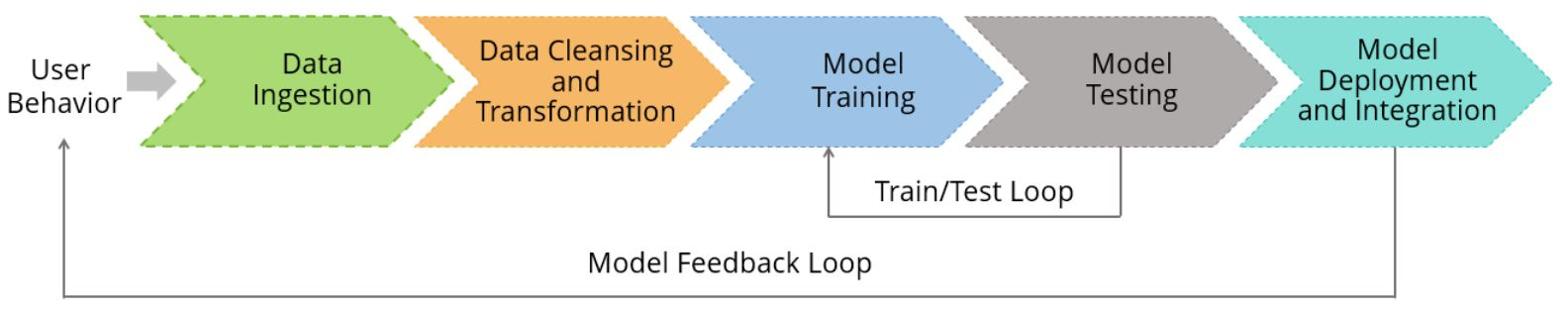In the next topic, you will learn about the three C's or the techniques for exploiting data.

## The Three C’s of Machine Learning

In the previous topic, you learned about the applications of machine learning and the role of apache spark machine learning. In this topic, you will learn about the three techniques for exploiting data.

Let’s take a look at what these three techniques are. These include:

• Collaborative filtering or recommendations

• Clustering

• Classification

You will learn more about them in the subsequent sections. Let’s begin with clustering.

### Clustering

Clustering is an unsupervised learning problem with the objective to group the subsets of entities by some idea of similarity.

It is generally used either as a hierarchical supervised learning pipeline component or for exploratory analysis.

MLlib supports k means clustering, which is a clustering machine learning algorithm. It clusters the data points into a predefined number of clusters. You will learn more about k means clustering in the sections ahead.

There are various other models of clustering that MLlib supports, such as Gaussian mixture, Power Iteration Clustering or PIC, Latent Dirichlet Allocation or LDA, and Streaming k means.

Let’s move on to classification.

### Classification

MLlib provides support to different regression analysis, binary classification, and multiclass classification methods. The table on the sections lists and explains the supported algorithms for every problem type.

 Problem Type Supported Method Binary Classification Linear SVM, logistic regression, decision trees, random forests, gradient-boosted trees, and Naive Bayes Multiclass Classifications logistic regression, decision trees, random forests, and Naive Bayes Regression linear least squares, Lasso, ridge regression, decision trees, random forests, gradient-boosted trees, and isotonic regression

Explanation of these methods is beyond the scope of this lesson. Let’s take a look at two examples of classification.

Examples of Classification

In the first example, you can see that the classification always gives a yes or no answer by creating routes.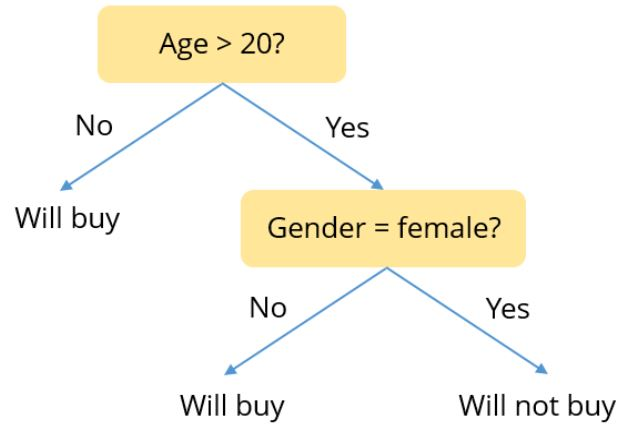In case of a certain product, if the age of the customer is more than 20, and if the customer is a female, it is likely that the customer will not buy the product.

The second example is a sample random graph for classification as shown below.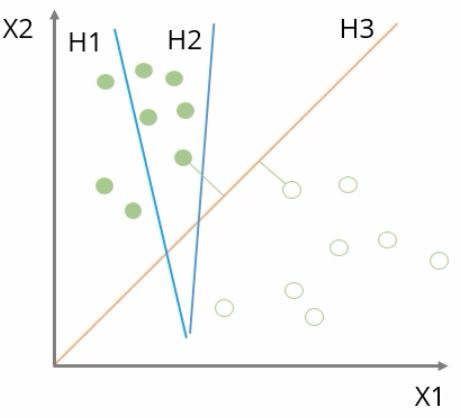### Collaborative Filtering

Collaborative filtering is generally used for recommender systems. The objective of these techniques is to complete a user-term association matrix with entries that are missing.

At present, MLlib provides support to model-based collaborative filtering. In this, products and users are explained through a small set of latent factors. These factors are used for predicting the missing entries.

The table below lists the parameters that facilitate the implementation of MLlib.

 Parameters Explanation numBlocks The number of blocks that are used for parallelizing computation rank The number of latent factors in the model Iteration The number of iterations to run lambda Parameter of regularization in ALS implicit Prefs Defines what should be used out of the variant adapted for implicit feedback data or the explicit feedback ALS variant alpha A parameter that is applicable to the implicit feedback ALS variant governing the baseline confidence in preference observation

## Machine Learning Challenges

Even though there are various techniques of exploiting data, which you have seen on the previous sections, there are some challenges in machine learning.

• Machine learning is highly computation intensive and iterative.

• There are many traditional numerical processing systems that do not scale to very large datasets. For example, MatLab.

• Machine learning seems to be disrupting software engineering, challenging its impact on real-world applications.

Now let’s move onto k means clustering which is a machine learning algorithm.

## K-Means Clustering: Machine learning Algorithm

You have learned that MLlib supports k means clustering, which is one of the most commonly used clustering algorithms.

K means clusters the data points into a predefined number of clusters. A parallelized variant of the K means++ method is also included, k means-pipeline-pipeline.

The table below lists the parameters that facilitate the implementation of MLlib.

 Parameters Explanation K The number of required clusters maxIterations The maximum number of iterations to run initializationMode Random initialization or initialization via the k means method runs The number of times to run the k means algorithm initialization Steps The number of steps in the k means algorithm epsilon The distance threshold within which you consider K means to have converged

Shown below is the flowchart that illustrates how the K means algorithm works.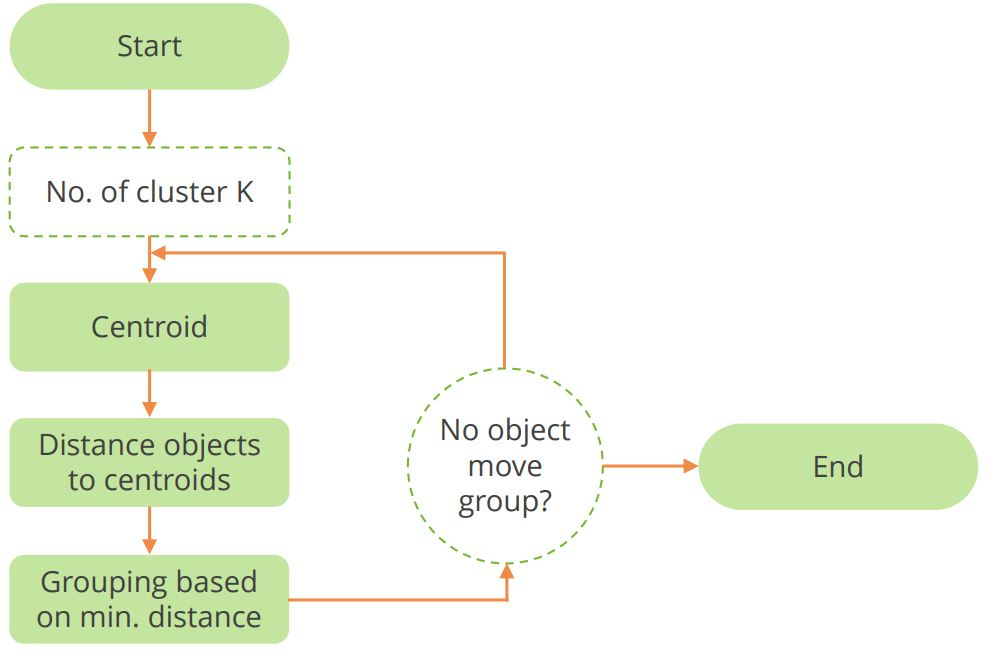## K-means at Work

To begin with, the k-means clustering algorithm tries to split a given anonymous data set, which contains no information as to class identity, into a fixed number (k) of clusters.

Initially, the k number of centroids, which is a data point, imaginary or real, at the center of a cluster, are chosen.

All centroids are unique, as each centroid is an existing data point in the given input data set, picked randomly. For each data point, you need to calculate the absolute distance between the point and each of the k centroids.

The data point then becomes part of the group of the centroid that matches the data points minimum distance.

Repeat steps 2 and five until the centroids no longer move. This produces a separation of the objects into groups from which the metric to be minimized can be calculated.

Now, let’s look at an example of k means machine learning example.

## K-means Clustering: Machine Learning Example

A telecommunications company wants to set up three towers to cover the entire population of a region and wants to understand how the customers are distributed. Here, you can see an anonymous data set.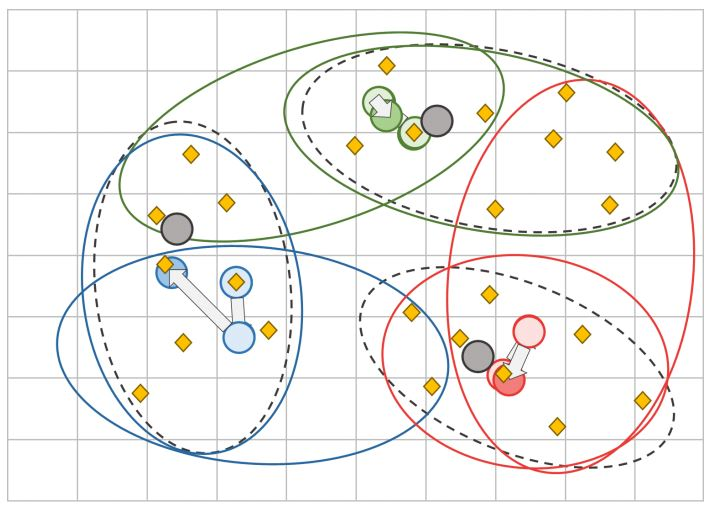The data needs to be clustered. The goal is to find the cluster of data points, using k-means clustering.

As the first step, choose K random points as starting centers.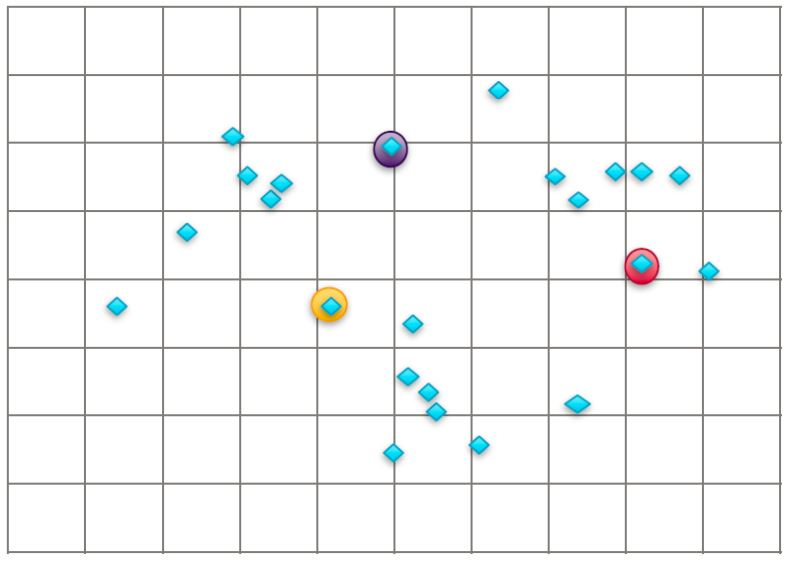As you can see, three random points are chosen. These indicate the location where the three towers will be located.

Secondly, find all points closest to each center. This is done by calculating the distance between the points.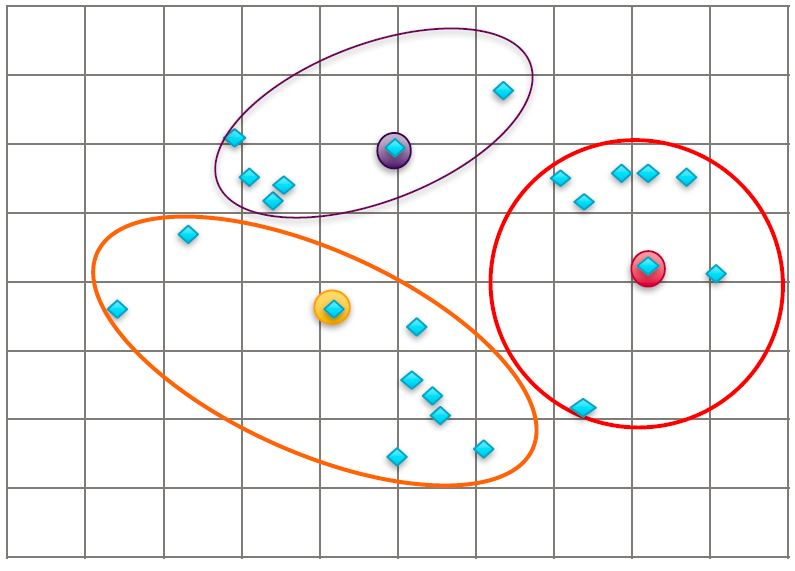For example, the data points closest to the yellow point are clustered. Then find the center or the mean of each cluster. If the centers change, iterate again.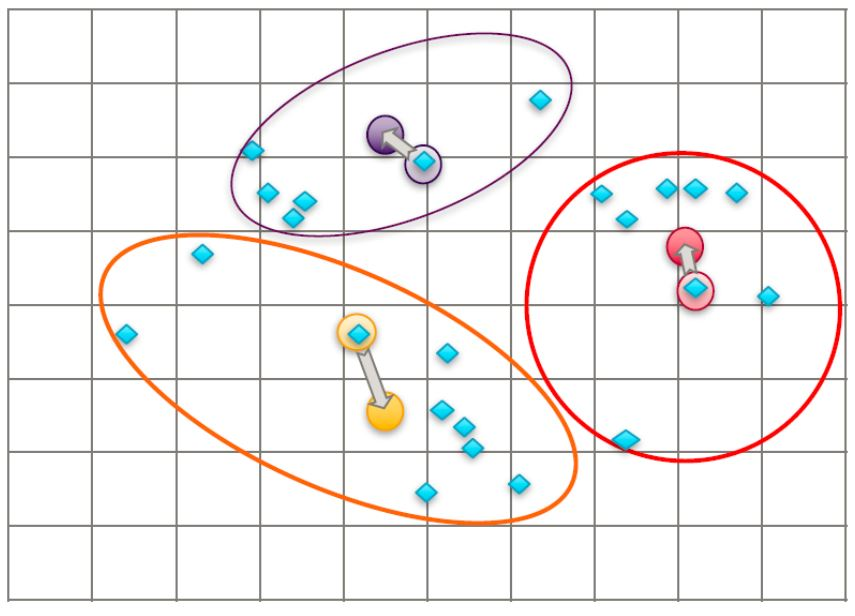You need to go back to step 2 to find all points that are closest to each center. These will look different compared to the points in step Again, repeat the earlier step 3 of finding the center or mean of each cluster.

If you find that the centers change again, you need to iterate again. Continue to do this until the centroids no longer move. You are finally done.

## Summary

Let’s now summarize what we learned in this lesson.

• Spark is designed for systems that are required to implement the iterative algorithm, that is, PageRank Algorithm, which is one of the methods used to determine a page's relevance or importance.

• Spark GraphX is a graph computation system running in the framework of the data-parallel system, which focuses on distributing the data across different nodes that operate on the data in parallel.

• Machine learning is a subfield of Artificial Intelligence or AI that deals with the construction and study of systems that can learn from data.

• The scalable Machine Learning library of Spark is called MLlib that contains general learning utilities and algorithms, including regression, collaborative filtering, classification, clustering, dimensionality reduction, and underlying optimization primitives.

• Clustering is an unsupervised learning problem with the objective to group the subsets of entities by some idea of similarity. It is generally used either as a hierarchical supervised learning pipeline component or for exploratory analysis.

• MLlib provides support to different regression analysis, binary classification, and multiclass classification methods.

• Collaborative filtering is generally used for recommender systems. The objective of these techniques is to complete a user-term association matrix with entries that are missing.

• MLlib supports k-means clustering, one of the most commonly used machine learning algorithms. K-means clusters the data points into a predefined number of clusters.

## Conclusion

This concludes the lesson “Spark Algorithm.” In the next lesson, we will talk about Spark SQL.

### Find our Big Data Hadoop and Spark Developer Online Classroom training classes in top cities:

Name Date Place
Big Data Hadoop and Spark Developer 23 Apr -4 Jun 2021, Weekdays batch Your City View Details
Big Data Hadoop and Spark Developer 1 May -12 Jun 2021, Weekend batch Dallas View Details
Big Data Hadoop and Spark Developer 10 May -1 Jun 2021, Weekdays batch New York City View Details
Related Courses
Learner Reviews
Related Articles
• Disclaimer
• PMP, PMI, PMBOK, CAPM, PgMP, PfMP, ACP, PBA, RMP, SP, and OPM3 are registered marks of the Project Management Institute, Inc.

#### Request more information

###### For business
Name*
Email*
Phone Number*
Your Message (Optional)
###### Our consultants will get in touch with you soon.

A Simplilearn representative will get back to you in one business day.

First Name*
Last Name*
Work Email*
Phone Number*
Company*
Job Title*